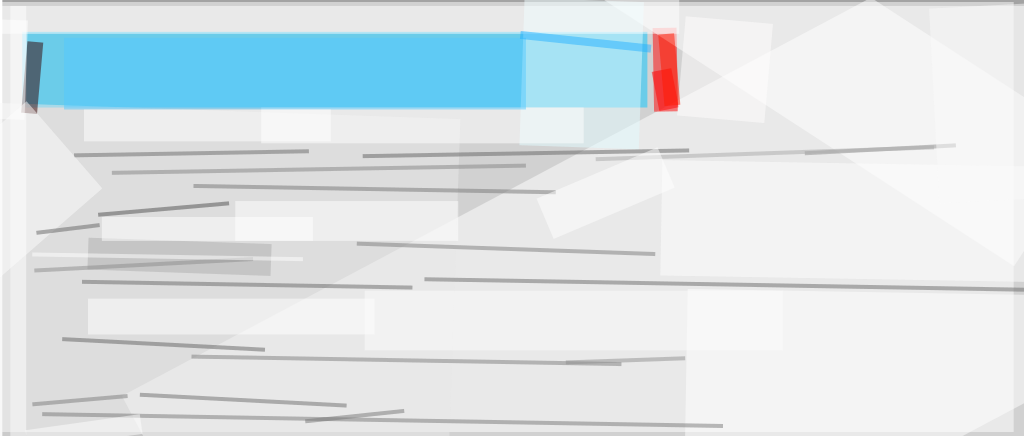# Templating in d3js

Let’s say you created a vector image such as this bar chart:Now you like to put this visualization on the web and run it on dynamic data. This tutorial covers how to achieve this by using ICanHaz templating in D3.js. All files for this tutorial can be found on GitHub.

## Why Using Templates

Creating simple bar charts in d3.js is very easy:

```<svg width="550px" height="0px">

```

The result should look like this: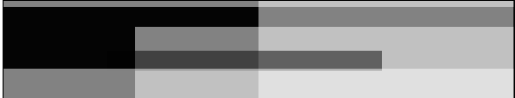So why not use this approach to bring our visualization to life? The above chart is based on single rectangles. Our design, on the other hand, has five different elements per bar: a black and red marker at the start and end respectively, the blue bar, the label and the gradient effect. We could of course append those five elements one by one with a sequence of append statements; and set the properties with attr. I dislike doing this for the following reasons:

1. The resulting code is not very readable
2. The d3 code will soon contain more presentation elements than actual code
3. You need to do cumbersome translations of you vector graphic into d3 method chains

Templating allows you to separate the design of visual from the rest of the d3 code.

## Creating the SVG Bars

Before going into JavaScript, first design your visualization. You can certainly do this directly in SVG, but a vector editor is probably more suitable for the task. I used Adobe Illustrator to design the bar from this tutorial …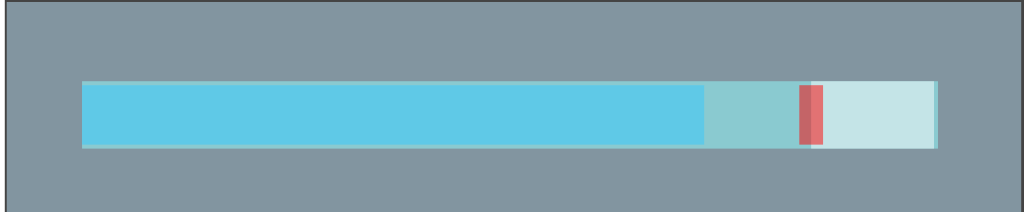… and exported it to SVG with the default settings:The resulting SVG looks like that:

```<?xml version="1.0" encoding="utf-8"?>
<!-- Generator: Adobe Illustrator 16.0.0, SVG Export Plug-In . SVG Version: 6.00 Build 0)  -->
<!DOCTYPE svg PUBLIC "-//W3C//DTD SVG 1.1//EN" "http://www.w3.org/Graphics/SVG/1.1/DTD/svg11.dtd">
width="200px" height="16px" viewBox="0 0 200 16" enable-background="new 0 0 200 16" xml:space="preserve">
<stop  offset="0" style="stop-color:#78D2FF"/>
<stop  offset="1" style="stop-color:#24B6FF"/>
<rect y="1" fill="url(#SVGID_1_)" width="173" height="14"/>
<g>
<text transform="matrix(0.9756 0 0 1 143 13)" fill="#FFFFFF" font-family="'Exo-DemiBold'" font-size="13">200</text>
</g>
<rect x="168" y="1" fill="#FF2312" width="5" height="14"/>
<rect y="1" width="2" height="14"/>
<rect y="1" opacity="0.2" fill="#FFFFFF" width="173" height="6"/>
</svg>
```

The raw output is then simplified by applying the following steps:

• remove the dispensable lines, i.e., the xml declaration statement, the adobe illustrator comment, the <!Doctype> declaration and the svg opening tag and closing tag.
• remove the group around the single element.
• replace the transform=”matrix(0.9756 0 0 1 143 13)” with the equivalent, but more readable, transform=”translate(143 13)”
• Important: the SVG might not render at all in Safari if the linear gradient is not defined inside defs tags.

After cleanup we get this SVG snippet:

```<defs>
<stop  offset="0" style="stop-color:#78D2FF"/>
<stop  offset="1" style="stop-color:#24B6FF"/>
</defs>
<rect y="1" fill="url(#SVGID_1_)" width="173" height="14"/>
<text transform="translate(143 13)" fill="#FFFFFF" font-family="'Exo-DemiBold'" font-size="13">200</text>
<rect x="168" y="1" fill="#FF2312" width="5" height="14"/>
<rect y="1" width="2" height="14"/>
<rect y="1" opacity="0.2" fill="#FFFFFF" width="173" height="6"/>
```

## Using the SVG Bars in D3.js

Three javascript libraries are required in addition to d3.js to make templating work:

• The templating library ICanHaz.
• JQuery to check when the dom, and the template in particular, is ready
• For reasons explained later on also need the small innersvg library.
```<script type="text/javascript" src="scripts/d3.v3.js"></script>
<script type="text/javascript" src="scripts/ICanHaz.min.js" ></script>
<script type="text/javascript" src="scripts/jquery-1.10.2.min.js"></script>
<script type="text/javascript" src="scripts/innersvg.js"></script>```

After that, we put an empty svg where we want the bar chart to appear:

`<svg width="920" height="0"></svg>`

Next we put the template inside script tags. The id attribute is used to reference the template later on.

```<script id="row" type="text/html">
<defs>
<stop  offset="0" style="stop-color:#78D2FF"/>
<stop  offset="1" style="stop-color:#24B6FF"/>
</defs>
<rect y="1" fill="url(#SVGID_1_)" width="173" height="14"/>
<text transform="translate(143 13)" fill="#FFFFFF" font-family="'Exo-DemiBold'" font-size="13">200</text>
<rect x="168" y="1" fill="#FF2312" width="5" height="14"/>
<rect y="1" width="2" height="14"/>
<rect y="1" opacity="0.2" fill="#FFFFFF" width="173" height="6"/>
</script>
```

Finally, we need to make small changes to the d3 part of the code. The first few lines remain the same:

```<script>
data = [{'value':200}, {'value':100}, {'value':150}, {'value':50}]
var x = d3.scale.linear()
.domain([0, 200])
.range([0, 500]);

var rowHeight = 22;```

To ensure that the template is ready to be used, we put the whole d3.select… method chain inside \$(document).ready(function () {}. The height of the SVG is set as before:

```\$(document).ready(function () {
var svg = d3.select('svg')
.attr("height", rowHeight*data.length)
```

The bars are no longer simple rectangles, but consist of a couple of SVG lines. We add SVG groups with class dataRow as placeholder, which will later be filled with our template. So .selectAll(“rect”) becomes .selectAll(‘g.dataRow’), and instead of appending rect we append g and set the class name with .attr(“class”, “dataRow”):

```.selectAll('g.dataRow')
.data(data)
.enter()
.append('g')
.attr("class", "dataRow")```

To get the bars vertically stacked we translate the dataRow groups with

```.attr('transform', function(d,i) {
return "translate(0," + i*rowHeight + ")";
})
```

And, finally, we add our row template with:

```.html(function(d,i) {
return ich.row(d, true);
});```

Important: .html adds the template code to the innerHTML property which does not exists for SVG. This is where innersvg.js comes into play. The library adds the innerHTML property to SVG. Without this library, the code will probably still work in Google Chrome, but fail on most other browsers.

You can see the final code under `3_using_the_bars_in_d3.js/index.html`), it should produce this image: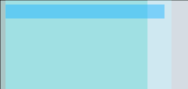If you look at the source code, you’ll see that the gradient is defined for each bar anew. Let’s fix this by moving the definition to the svg placeholder like this:

```<svg width="920" height="0">
<defs>
<stop  offset="0" style="stop-color:#78D2FF"/>
<stop  offset="1" style="stop-color:#24B6FF"/>
</defs>
</svg>

<script id="row" type="text/html">
<rect y="1" fill="url(#SVGID_1_)" width="173" height="14"/>
<text transform="translate(143 13)" fill="#FFFFFF" font-family="'Exo-DemiBold'" font-size="13">200</text>
<rect x="168" y="1" fill="#FF2312" width="5" height="14"/>
<rect y="1" width="2" height="14"/>
<rect y="1" opacity="0.2" fill="#FFFFFF" width="173" height="6"/>
</script>```

## Making the SVG Bars Dynamic

So far our code successfully loads and uses the template, but there isn’t any dynamic property yet. The template already gets the data values passed here return ich.row(d, true); and it can access properties of the data object by putting the name inside double curly brackets. In our case {{value}} will be replaced with 200 for the first row, with 100 for the second row and so on.

### Dynamic Labels

A simple change from

`<text transform="translate(143 13)" fill="#FFFFFF" font-family="'Exo-DemiBold'" font-size="13">200</text>`

to

`<text transform="translate(143 13)" fill="#FFFFFF" font-family="'Exo-DemiBold'" font-size="13">{{value}}</text>`

will already produce correct data labels: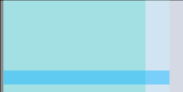(The full code after this step is `4_making_the_svg_bars_dynamic/step1.html`).

### Dynamic Bar Widths

The width of the bars is determined by the linear scale. ICanHaz-templates cannot evaluate functions, so instead we pass the value to the template as a new object property named width:

```.html(function(d,i) {
d = \$.extend(d,
{'width': x(d.value)})
return ich.row(d, true);
});```

We use this new property to set the length of the blue bar. Instead of the previously fixed width of 173 pixels we set:

`<rect y="1" opacity="0.2" fill="#FFFFFF" width="{{width}}" height="6"/>`

The same modification applies to the gradient effect:

`<rect y="1" fill="url(#SVGID_1_)" width="{{width}}" height="14"/>`

Those two changes will lead to this version of the bar chart: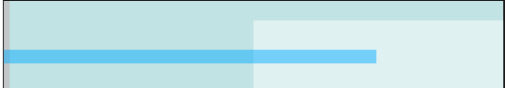(The full code after this step is `4_making_the_svg_bars_dynamic/step2.html`).

### Dynamic Label Position and End of Bar Marker

Finally, we need to set the position of the label and the red marker. Those two elements should be positioned relative to the end of the bar. The text element is currently at x-position143. Remember that the bar length in the SVG export was 173 pixels which means the text element is -30 pixels from the end of the bar. Similarly, the red marker is placed at 168 which corresponds to an offset of -5.

We could, of course, just calculate two new properties for those two positions and pass them to the template, e.g.:

```.html(function(d,i) {
d = \$.extend(d,
{'width': x(d.value)
'label_pos': x(d.value)-30,
'marker_pos': x(d.value)-5
});
return ich.row(d, true);
});```

But a more readable solution is to define the offset in SVG by grouping the text and marker. The group is positioned at the end of the bar, while the text is placed relative to this group at -30px and the marker at -5px.

```<script id="row" type="text/html">
<rect y="1" fill="url(#SVGID_1_)" width="{{width}}" height="14"/>
<g transform="translate({{width}} 0)">
<text transform="translate(-30 13)" fill="#FFFFFF" font-family="'Exo-DemiBold'" font-size="13">{{value}}</text>
<rect x="-5" y="1" fill="#FF2312" width="5" height="14"/>
</g>
<rect y="1" width="2" height="14"/>
<rect y="1" opacity="0.2" fill="#FFFFFF" width="{{width}}" height="6"/>
</script>```

After this last modification we get the desired result: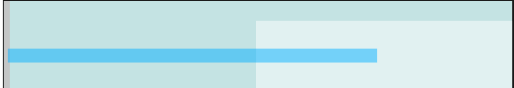(The final code is `4_making_the_svg_bars_dynamic/step3.html`).